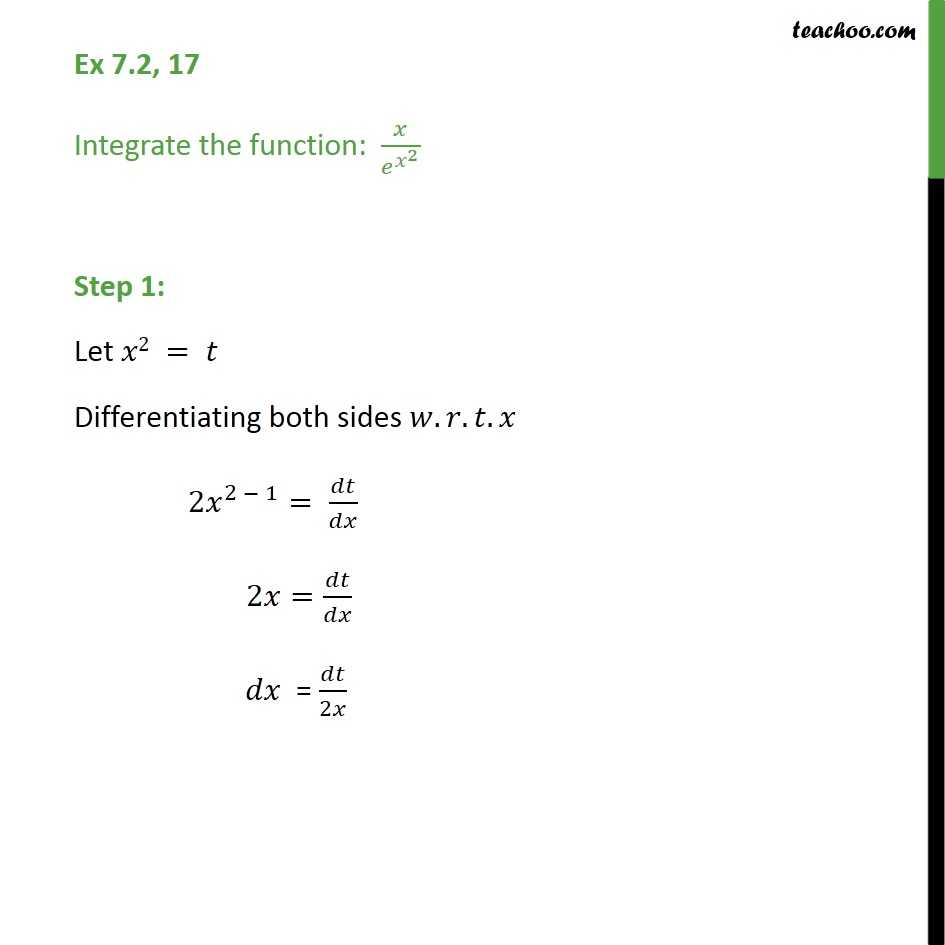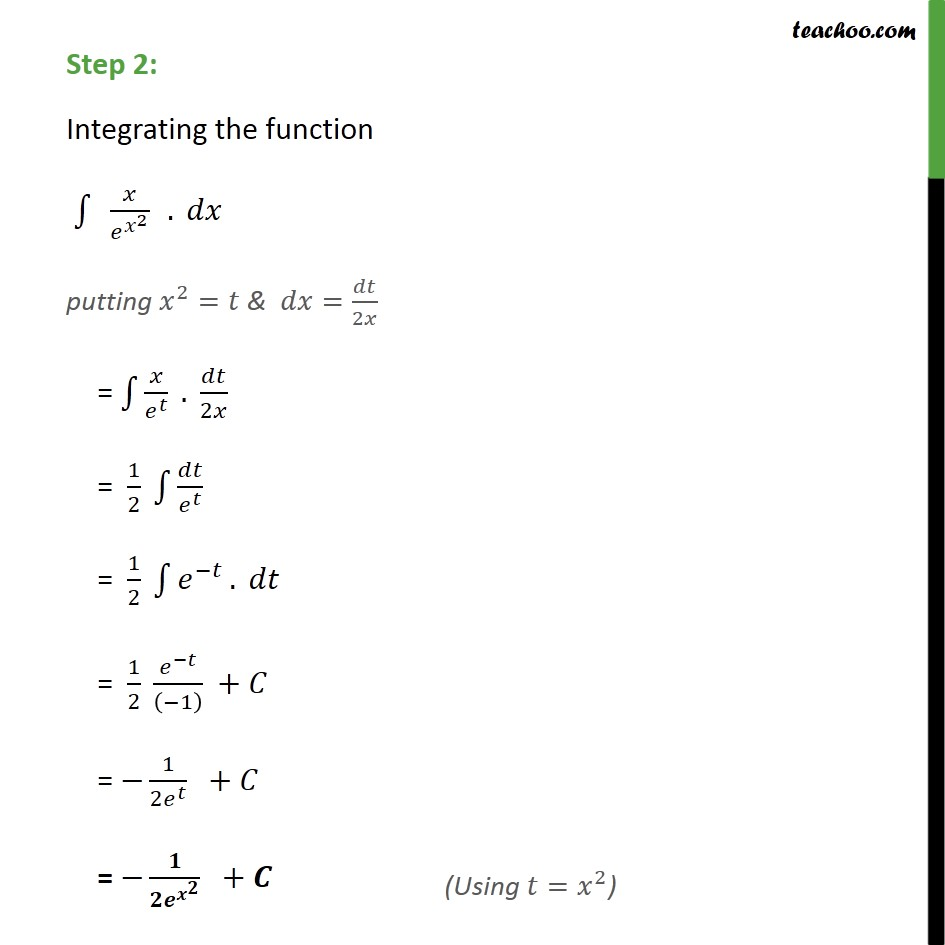1. Chapter 7 Class 12 Integrals (Term 2)
2. Serial order wise
3. Ex 7.2

Transcript

Ex 7.2, 17 Integrate the function: / ^( ^2 ) Step 1: Let 2 = Differentiating both sides . . . 2 ^(2 1)= / 2 = / " " = /2 Step 2: Integrating the function 1 " " / ^( ^2 ) " " . putting ^2= & = /2 = 1 / ^ . /2 = 1/2 1 / ^ = 1/2 1 ^( ) . = 1/2 ^( )/(( 1) ) + = 1/(2 ^ ) + = /( ^( ^ ) ) +

Ex 7.2